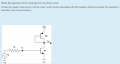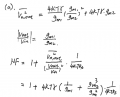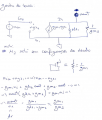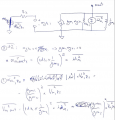# Obtain the expression of the noise figure for this circuit

#### asdasd12e12

Joined Nov 24, 2021
48
Here is the circuit:Here is the solution (taken from the book RF microelectronics by Behzad Razavi):My attempt:

First i tried to find the gain expression for this circuit, i noticed that M2 is in diode configuration so in the small signal model M2 is equivalent to a resistor (= 1/gm2).

I would say everything is ok here as i got the same gain as the solution.Now for the total noise at the output:The noise i got at the output by the contribuition of IdM1 is not the same as the solution.

In the solution it's Id^2 * gm2 while i got Id^2 * 1/gm2 . Also in the solution there's a term somewhat related to the Vm1,Rs noise i computed but instead it has a id^2/gm1 factor. I dont know where that came from.

What am i doing wrong here?